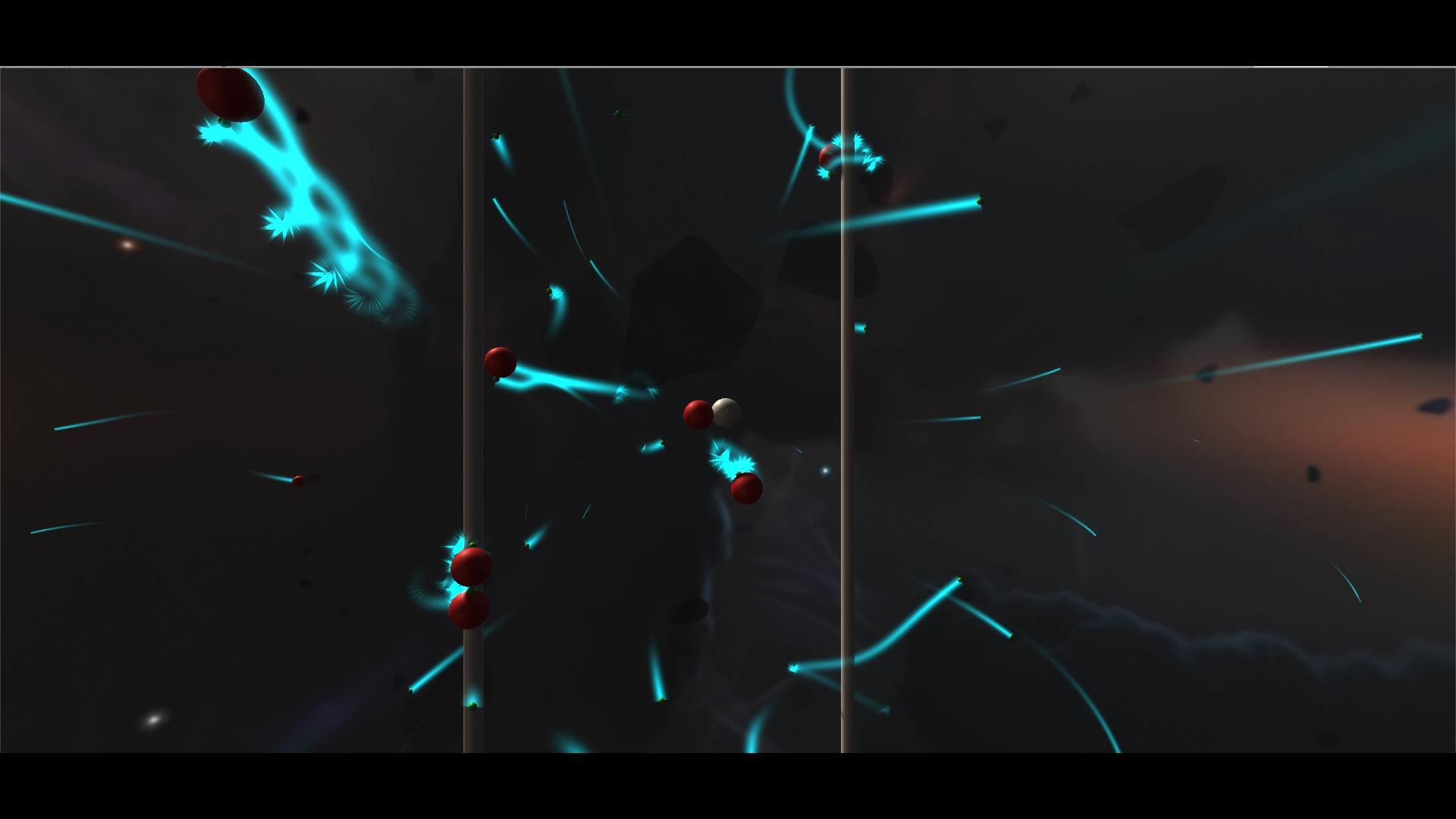CREATING A 3D ELECTROMAGNETIC FIELD
INTRODUCTION

In this tutorial I will be explaining how to create an electromagnetic simulator and understand the maths and physics we use behind it. This tutorial is only an explanation where you will learn how to design your own simulator with the information you will see next.

The simulator I created have electrons and protons that interact between them and also with the conductors. These conductors will create a magnetic field that electrons and protons will be interacting with.

Here are the contents we will be touching:

• Understanding the project
• Structure of the project
• Electric field
• Magnetic field
• Combining both fields
UNDERSTANDING THE PROJECT

Protons and electrons interacts between them (electric field).They will interact also with conductors that creates a magnetic field. You will see how this two forces interact and the electrons will describe helicoidal trayectories and orbits.

I used some formulas but the constants I used are not real. This is because if you add to an electron an speed of 20000m/s you won't see it on screen

If you have watched you will have noticed that the simulator is in a 3D View. This is the reason why we will be using vectorized notations because we can't work only with modulus.

STRUCTURE OF PROJECT

I have created different classes in C# for the project. This is only an example on how you can manage your simulator

• Electric Handler
• Charge
• Camera
• Conductor
ELECTRIC HANDLER

Is in charge of spawning the electrons and protons and displaying the conductors. It will carry all the settings for the simulator

It will have variables such us the spawn rate, maximum distance to spawn, impulse, proportion of size between electrons and protons, probability of spawning an electron or a proton, initial speed of electrons and the maximum of particles on screen

It will control also when to create and destroy each component

CHARGE

Depending if it's a proton or an electron it will have different charge value, material and size. We will add here all the math and physics interaction for every charge.

If it's a proton it will have a positive charge and if it's an electron it will have a negative charge. It will execute all the math and physics formulas

We will need to apply the superposition and interact with every charge. So we will need to make a foreach through every charge in each charge

CAMERA

The camera will rotate around the spawn point

CONDUCTOR

The conductors will only have the variables intensity and permeability. The math will be executed on each charge.

ELECTRIC FIELD

The program integrate an electric simulator that works with the vectorized Coloumb Law. This law works as the Universal Gravity of Law but it could be repulsive or attractive and it will depend on the charges

d is the distance between the two charges and q is the value of the charge

$$F_e = K\frac{q_1q_2}{r^2}\vec{u_r}$$

K is the Coloumb Constant and is equal to:

$$K=\frac{1}{4\pi\epsilon_0}$$

The demonstration of the Coloumb's Law is using the Gauss Law:

$$\oint_V{\vec{E}·\vec{dA}}=\frac{Q_{int}}{\epsilon_0}$$

Coulomb’s Law relates force to charge and Gauss’s Law relates electric field to charge so that looks like the equation to use. Enclose the single charge q in a sphere of radius r. Because of symmetry, the electric field will be the same everywhere on the sphere’s surface. The left hand side of Gauss’s Law then reads:

$$\oint{\vec{E}·\vec{dA}}=E·\oint{\vec{dA}}=E·A=E4\pi r^2$$

becuase the area of a sphere is $$4\pi r^2$$.

Then we will get $$E$$ with the Gauss Theorem knowing that $$Q_{int}=q$$

$$E4\pi r^2=\frac{Q_{int}}{\epsilon_0}\Rightarrow E = \frac{1}{4\pi \epsilon_0}\frac{q}{r^2}=K\frac{q}{r^2}$$

$$E$$ is the intensity of the electric field. If we want the force $$F_e=q·E$$

Then we will apply the superposition and get the force from the other charges in world. For example if we want to get the force of charge 1 we must get the electric force between charges 2 and 3 to charge 1:

$$F_1=F_{21}+F_{31}$$

MAGNETIC FIELD

There is also a magnetic field because of the electrical conductors with intensity I

We use the Lorentz Law to get the force depending on the intensity of the conductors and the induction of magnetic field $$\vec{B}$$

$$F_m=q(\vec{v}\times\vec{B})$$

The second Lorentz law is for getting the force of a conductor to another:

$$\vec{F_m}=I(\vec{l}\times\vec{B})$$

We should know the magnetic field induction. In order to get it we can use the formula of Biot and Savat that with the intensity,permeability, the direction of the conductor and the position vector of the charge we can get $$\vec{B}$$

$$\vec{B}=\frac{\mu_0 I}{2\pi r}(\vec{u_l}\times\vec{u_r})$$

If there are more conductors that are interacts we must use another formula:

$$\vec{B}=\frac{\mu_0 I_1 I_2}{2\pi r}(\vec{u_l}\times\vec{u_r})$$

If you want to know information about the circular trayectories of the particles:

$$R=\frac{mv}{qB}\Rightarrow T=\frac{2\pi m}{q B}$$

ELECTROMAGNETIC FIELD

When you create both fields you can now get the finl force to apply to an specific particle. It will be the sum of both forces. Then with the mass you will get the acceleration and executed a MRUA trayectory

$$\vec{F}=\vec{F_e}+\vec{F_m}$$